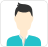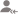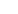Find the best tutors and institutes for Class 12 Tuition

Find Best Class 12 Tuition

Please select a Category.

Please select a Locality.

No matching category found.

No matching Locality found.

Outside India?

Search for topics# Class 12 Tuition Updates

Post a Lesson

Answered on 13 Sep CBSE/Class 12/Mathematics/Continuity and Differentiability/NCERT Solutions/Exercise 5.1

Prove that the functionis continuous at read more

Prove that the functionis continuous atread lessSwapna Shree

Therefore, f is continuous at x = 0 Therefore, f is continuous at x = −3 Therefore, f is continuous at x = 5 read moreTherefore, f is continuous at x = 0Therefore, f is continuous at x = −3Therefore, f is continuous at x = 5

Dislike Bookmark

Answered on 13 Sep CBSE/Class 12/Mathematics/Continuity and Differentiability/NCERT Solutions/Exercise 5.1

Examine the continuity of the function. read more

Examine the continuity of the function. read lessSwapna Shree

Thus, f is continuous at x = 3 read moreThus, f is continuous at x = 3

Dislike Bookmark

Answered on 13 Sep CBSE/Class 12/Mathematics/Continuity and Differentiability/NCERT Solutions/Exercise 5.1

Examine the following functions for continuity. (a) (b) (c) (d) read more

Examine the following functions for continuity.

(a)(b)(c)(d)read lessSwapna Shree

(a) The given function is It is evident that f is defined at every real number k and its value at k is k − 5. It is also observed that, Hence, f is continuous at every real number and therefore, it is a continuous function. (b) The given function is For any real number k ≠ 5, we obtain Hence,... read more

(a) The given function isIt is evident that f is defined at every real number k and its value at k is k − 5.

It is also observed that,Hence, f is continuous at every real number and therefore, it is a continuous function.

(b) The given function isFor any real number k ≠ 5, we obtainHence, f is continuous at every point in the domain of f and therefore, it is a continuous function.

(c) The given function isFor any real number c ≠ −5, we obtainHence, f is continuous at every point in the domain of f and therefore, it is a continuous function.

(d) The given function isThis function f is defined at all points of the real line.

Let c be a point on a real line. Then, c < 5 or c = 5 or c > 5

Case I: c < 5

Then, f (c) = 5 − cTherefore, f is continuous at all real numbers less than 5.

Case II : c = 5

Then,Therefore, f is continuous at x = 5

Case III: c > 5Therefore, f is continuous at all real numbers greater than 5.

Hence, f is continuous at every real number and therefore, it is a continuous function.

Dislike Bookmark

Looking for Class 12 Tuition

Find best Class 12 Tuition in your locality on UrbanPro.

FIND NOW

Answered on 13 Sep CBSE/Class 12/Mathematics/Continuity and Differentiability/NCERT Solutions/Exercise 5.1

Find all points of discontinuity of f, where f is defined by read more

Find all points of discontinuity of f, where f is defined byread lessSwapna Shree

The given function f is The given function f is defined at all the points of the real line. Let c be a point on the real line. Case I: Therefore, f is continuous at all points x, such that x < 1 Case II: If c = 1, then the left hand limit of f at x = 1 is, The right hand limit of f at x = 1 is, It... read more

The given function f isThe given function f is defined at all the points of the real line.

Let c be a point on the real line.

Case I:Therefore, f is continuous at all points x, such that x < 1

Case II:

If c = 1, then the left hand limit of f at x = 1 is,The right hand limit of f at x = 1 is,It is observed that the left and right hand limit of f at x = 1 do not coincide.

Therefore, f is not continuous at x = 1

Case III:Therefore, f is continuous at all points x, such that x > 1

Thus, from the above observation, it can be concluded that x = 1 is the only point of discontinuity of f.

Dislike Bookmark

Answered on 13 Sep CBSE/Class 12/Mathematics/Continuity and Differentiability/NCERT Solutions/Exercise 5.1

Is the function defined by a continuous function? read more

Is the function defined bya continuous function? read lessSwapna Shree

The given function is The given function f is defined at all the points of the real line. Let c be a point on the real line. Case I: Therefore, f is continuous at all points x, such that x < 1 Case II: The left hand limit of f at x = 1 is, The right hand limit of f at x = 1 is, It is observed... read more

The given function isThe given function f is defined at all the points of the real line.

Let c be a point on the real line.

Case I:Therefore, f is continuous at all points x, such that x < 1

Case II:The left hand limit of f at x = 1 is,The right hand limit of f at x = 1 is,It is observed that the left and right hand limit of f at x = 1 do not coincide.

Therefore, f is not continuous at x = 1

Case III:Therefore, f is continuous at all points x, such that x > 1

Thus, from the above observation, it can be concluded that x = 1 is the only point of discontinuity of f.

Dislike Bookmark

Answered on 13 Sep CBSE/Class 12/Mathematics/Continuity and Differentiability/NCERT Solutions/Exercise 5.1

Discuss the continuity of the function f, where f is defined by read more

Discuss the continuity of the function f, where f is defined byread lessSwapna Shree

The given function is The given function is defined at all points of the interval . Let c be a point in the interval . Case I: Therefore, f is continuous in the interval . Hence, f is not continuous at x = 1 and x = 3 read more

The given function isThe given function is defined at all points of the interval [0, 10].

Let c be a point in the interval [0, 10].

Case I:Therefore, f is continuous in the interval [0, 1).

Case II:The left hand limit of f at x = 1 is,The right hand limit of f at x = 1 is,It is observed that the left and right hand limits of f at x = 1 do not coincide.

Therefore, f is not continuous at x = 1

Case III:Therefore, f is continuous at all points of the interval (1, 3).

Case IV:The left hand limit of f at x = 3 is,The right hand limit of f at x = 3 is,It is observed that the left and right hand limits of f at x = 3 do not coincide.

Therefore, f is not continuous at x = 3

Case V:Therefore, f is continuous at all points of the interval (3, 10].

Hence, f is not continuous at x = 1 and x = 3

Dislike Bookmark

Answered on 13 Sep CBSE/Class 12/Mathematics/Continuity and Differentiability/NCERT Solutions/Exercise 5.1

Find the points of discontinuity of f, where read more

Find the points of discontinuity of f, whereread lessSwapna Shree

The given function f is It is evident that f is defined at all points of the real line. Let c be a real number. Case I: Therefore, f is continuous at all points x, such that x < 0 Case II: Therefore, f is continuous at all points x, such that x > 0 Case III: The left hand limit of f at x = 0... read more

The given function f isIt is evident that f is defined at all points of the real line.

Let c be a real number.

Case I:Therefore, f is continuous at all points x, such that x < 0

Case II:Therefore, f is continuous at all points x, such that x > 0

Case III:The left hand limit of f at x = 0 is,The right hand limit of f at x = 0 is,Therefore, f is continuous at x = 0

From the above observations, it can be concluded that f is continuous at all points of the real line.

Thus, f has no point of discontinuity.

Dislike Bookmark

Answered on 13 Sep CBSE/Class 12/Mathematics/Continuity and Differentiability/NCERT Solutions/Exercise 5.1

Determine if f defined by is a continuous function? read more

Determine if f defined byis a continuous function? read lessSwapna Shree

The given function f is It is evident that f is defined at all points of the real line. Let c be a real number. Case I: Therefore, f is continuous at all points x ≠ 0 Case II: ⇒−x2≤x2sin1x≤x2⇒-x2≤x2sin1x≤x2 Therefore, f is continuous at x = 0 From the above observations,... read more

The given function f isIt is evident that f is defined at all points of the real line.

Let c be a real number.

Case I:Therefore, f is continuous at all points x ≠ 0

Case II:x2x2sin1xx2⇒-x2≤x2sin1x≤x2Therefore, f is continuous at x = 0

From the above observations, it can be concluded that f is continuous at every point of the real line.

Thus, f is a continuous function.

Dislike Bookmark

Answered on 13 Sep CBSE/Class 12/Mathematics/Continuity and Differentiability/NCERT Solutions/Exercise 5.1

Examine the continuity of f, where f is defined by read more

Examine the continuity of f, where f is defined byread lessSwapna Shree

The given function f is It is evident that f is defined at all points of the real line. Let c be a real number. Case I: Therefore, f is continuous at all points x, such that x ≠ 0 Case II: Therefore, f is continuous at x = 0 From the above observations, it can be concluded that f is continuous... read more

The given function f isIt is evident that f is defined at all points of the real line.

Let c be a real number.

Case I:Therefore, f is continuous at all points x, such that x ≠ 0

Case II:Therefore, f is continuous at x = 0

From the above observations, it can be concluded that f is continuous at every point of the real line.

Thus, f is a continuous function.

Dislike Bookmark

Looking for Class 12 Tuition

Find best Class 12 Tuition in your locality on UrbanPro.

FIND NOW

Answered on 13 Sep CBSE/Class 12/Mathematics/Continuity and Differentiability/NCERT Solutions/Exercise 5.1Swapna Shree

This function f is defined for every real number and f can be written as the composition of two functions as, f = g o h, where It has to be proved first that are continuous functions. Clearly, g is defined for all real numbers. Let c be a real number. Case I: Therefore, g is continuous at all points... read moreThis function f is defined for every real number and f can be written as the composition of two functions as,

f = g o h, whereIt has to be proved first thatare continuous functions.Clearly, g is defined for all real numbers.

Let c be a real number.

Case I:Therefore, g is continuous at all points x, such that x < 0

Case II:Therefore, g is continuous at all points x, such that x > 0

Case III:Therefore, g is continuous at x = 0

From the above three observations, it can be concluded that g is continuous at all points.

h (x) = sin x

It is evident that h (x) = sin x is defined for every real number.

Let c be a real number. Put x = c + k

If xc, then k → 0

h (c) = sin cTherefore, h is a continuous function.

It is known that for real valued functions g and h,such that (g o h) is defined at c, if g is continuous at c and if f is continuous at g (c), then (f o g) is continuous at c.

Therefore,is a continuous function.

Dislike Bookmark

UrbanPro.com helps you to connect with the best Class 12 Tuition in India. Post Your Requirement today and get connected.

Overview

Questions 34

Total Shares17 Followers

x